# Samacheer Kalvi 9th Maths Solutions Chapter 7 Mensuration Additional Questions

## Tamilnadu Samacheer Kalvi 9th Maths Solutions Chapter 7 Mensuration Additional Questions

Exercise 7.1

Question 1.
Using Heron’s formula, find the area of a triangle whose sides are 41 m, 15 m, 25 m.
Solution: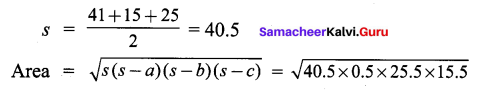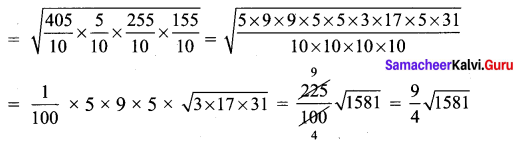Question 2.
Find the area of an equilateral triangle whose perimeter is 150 m.
Solution:
3a = 150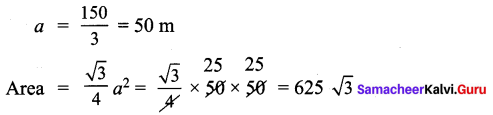Question 3.
Find the area of a quadrilateral whose sides are PQ = 15 cm, QR = 8 cm, RS = 25 cm, PS = 12 cm and LQ = 90°
Solution: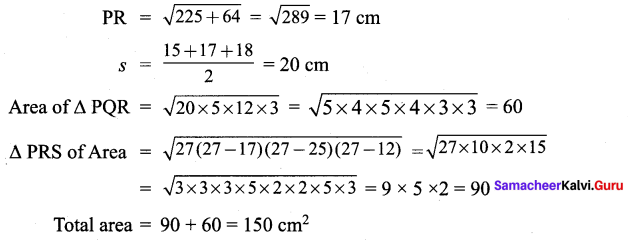Exercise 7.2

Question 1.
Find the TSA and LSA of a cuboid whose length, breadth and height are 10 cm, 12 cm and 14 cm respectively.
Solution:
TSA = 2 (lb + bh + Ih)
= 2 (10 × 12 + 12 × 14 + 10 × 14) = 2 (120 + 168 + 140) = 856 cm2
LSA = 2 (bh + Ih) = 2 (12 × 14 + 10 × 14) = 2 (168 + 140) =2 (308) = 616 cm2

Question 2.
A cuboid has total surface area of 40 m2 and its lateral surface area is 26 m2. Find the area of its base.
Solution: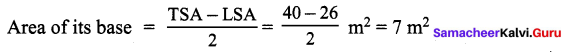Question 3.
Find the surface area of a cube whose edge is
(i) 27 cm
(ii) 3 cm
(iii) 6 cm
(iv) 2.1 cm
Solution:
(i) TSA = 6a2 = 6 × 272 = 6 × 729 = 4374 cm2
(ii) TSA = 6a2 = 6 × 32 = 54 cm2
(iii) TSA = 6a2 = 6 × 62 = 6 × 36 = 216 cm2
(iv) TSA = 6a2 = 6 × 2.12 = 6 × 4.41 = 26.46 cm2

Exercise 7.3Question 1.
Find the volume of a cube whose surface area is a 96 cm2.
Solution:
6a2 = 96 cm2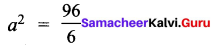a = 6
Volume = a3 = 63 = 36 × 6 = 216 cm3

Question 2.
The volume of a cuboid is 440 cm3 and the area of its base is 88 cm2, find its height.
Solution:
l × b × h = 440 cm3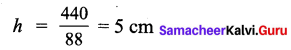Question 3.
How many 3 metre cubes can be cut from a cuboid measuring 18 m × 12 m × 9 m?
Solution: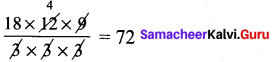Question 4.
The outer dimensions of a closed wooden box are 10 cm by 8 cm by 7 cm. Thickness
of the wood is 1 cm3. Find the total cost of wood required to make box if 1 cm3 of wood costs ₹ 2.00?
Solution:
Volume of wood = 12 × 10 × 9 – 10 × 8 × 7 = 1080 – 560 = 520 cm3 × 2.00 = ₹ 1040